Homework Help Question & Answers

# 1. A production function is given by f(K, L) = L/2+ v K. Given this form,...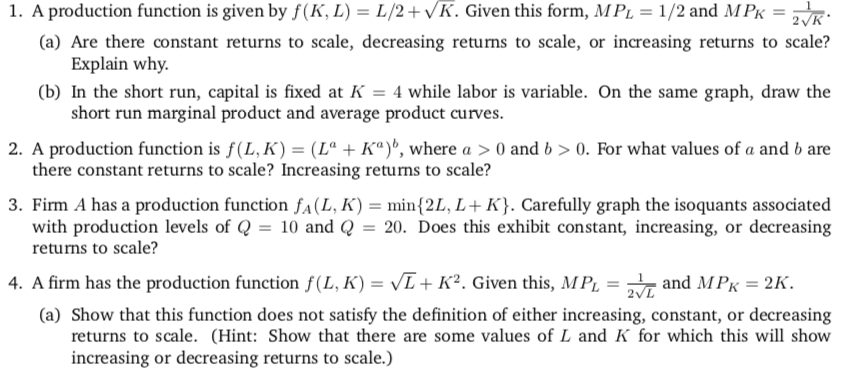1. A production function is given by f(K, L) = L/2+ v K. Given this form, MPL = 1/2 and MPK-2 K (a) Are there constant returns to scale, decreasing returns to scale, or increasing returns to scale? (b) In the short run, capital is fixed at -4 while labor is variable. On the same graph, draw the 2. A production function is f(LK)-(L" + Ka)", where a > 0 and b > 0, For what values of a and b are 3. Firm A has a production function fA(L, K) = min(2L, L + K). Carefully graph the isoquants associated Explain why. short run marginal product and average product curves. there constant returns to scale? Increasing returns to scale? with production levels of Q- 10 and returns to scale? 20. Does this exhibit constant, increasing, or decreasing 4. A firm has the production function f(L, K)-vL+ K. Given this, MPL = 2 T and MPK = 2K. (a) Show that this function does not satisfy the definition of either increasing, constant, or decreasing returns to scale. (Hint: Show that there are some values of L and K for which this will show increasing or decreasing returns to scale.)

#### Homework Answers

Answer #1

(1)

Q = f(K, L) = (L/2) + (K)0.5

(a)

When both inputs are doubled, new production function becomes

Q* = (2L/2) + (2K)0.5 = (2L/2) + (2)0.5 x (K)0.5

The first component of production function (L/2) is exactly doubled, but since (2)0.5 < 2, the second component of production function is less than doubled. Therefore, output is less than doubled. Since doubling both inputs less than doubles the output, there are decreasing returns to scale.

(b)

When K = 4,

Q = (L/2) + (4)0.5 = (L/2) + 2

Marginal product (MPL) = dQ/dL = 1/2 = 0.5

Average product (APL) = Q/L = (1/2) + (2/L) = 0.5 + 2/L

Data table used for graph:

 L MPL APL 1 0.5 2.5 5 0.5 0.9 10 0.5 0.70 15 0.5 0.63 20 0.5 0.6 25 0.5 0.58 30 0.5 0.57 35 0.5 0.56 40 0.5 0.55 45 0.5 0.54 50 0.5 0.54

Graph: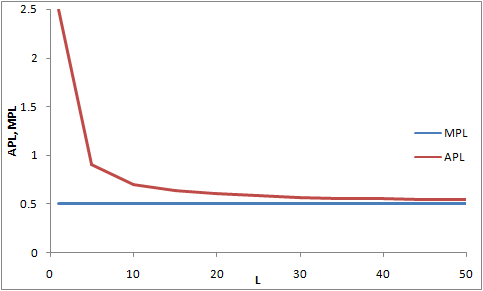NOTE: As per Answering Policy, 1st question is answered.

Know the answer?
Your Answer:

#### Post as a guest

Your Name:

What's your source?

#### Earn Coin

Coins can be redeemed for fabulous gifts.

Not the answer you're looking for? Ask your own homework help question. Our experts will answer your question WITHIN MINUTES for Free.
Similar Homework Help Questions
• ### Suppose the firm's production function is given by f(K,L) = min {K",L"} (a) For what values...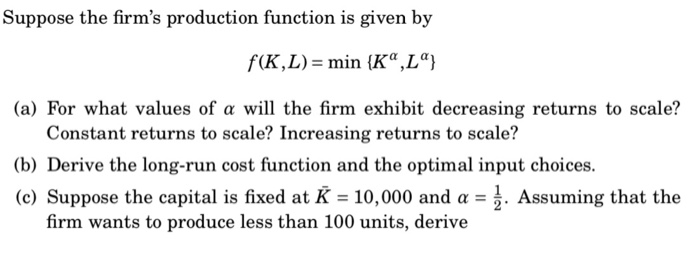Suppose the firm's production function is given by f(K,L) = min {K",L"} (a) For what values of a will the firm exhibit decreasing returns to scale? Constant returns to scale? Increasing returns to scale? (b) Derive the long-run cost function and the optimal input choices. (c) Suppose the capital is fixed at K = 10,000 and a = 1. Assuming that the firm wants to produce less than 100 units, derive

• ### A firm discovers that when it uses K units of capital and L units of labor,...

A firm discovers that when it uses K units of capital and L units of labor, it is able to produce X = L^1/4*K^3/4 units of output. a. Draw the graph of isoquants in labor-capital plane. b. Suppose that the firm produces 24 units of output using 16 units of capital and 81 units of labor. Compute MRTS subscript LK. Compute the MPL. Compute the MPK. c. On the basis of your answer to part (b), is the equation MRTS...

• ### 9. Suppose the firm's production function is given by f(K,L) min (K",L" (a) For what values...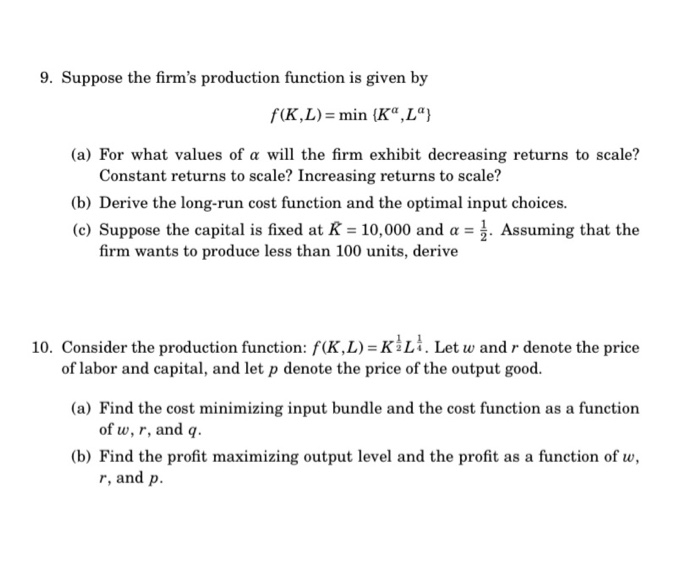9. Suppose the firm's production function is given by f(K,L) min (K",L" (a) For what values of a will the firm exhibit decreasing returns to scale? Constant returns to scale? Increasing returns to scale? (b) Derive the long-run cost function and the optimal input choices. (c) Suppose the capital is fixed at R = 10,000 and a =. Assuming that the firm wants to produce less than 100 units, derive 10. Consider the production function: f(K, L) = KLi. Let...

• ### 9. Suppose the firm's production function is given by f(K,L) = min (Kº,L"} (a) For what values of a will the firm e...9. Suppose the firm's production function is given by f(K,L) = min (Kº,L"} (a) For what values of a will the firm exhibit decreasing returns to scale? Constant returns to scale? Increasing returns to scale? (b) Derive the long-run cost function and the optimal input choices. (c) Suppose the capital is fixed at K = 10,000 and a = 1. Assuming that the firm wants to produce less than 100 units, derive 10. Consider the production function: f(K,L)=KLI. Let w...

• ### 1. Graph the short-run total product curves for each of the following production functions if K...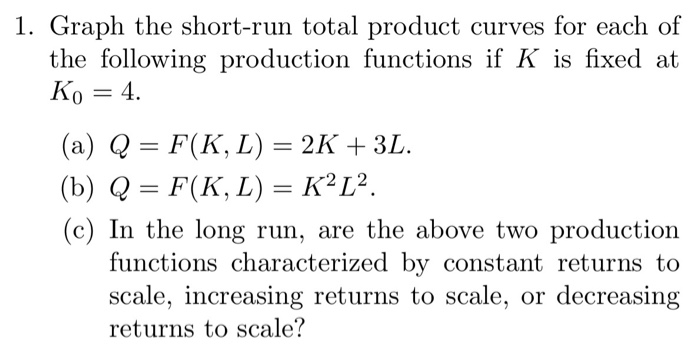1. Graph the short-run total product curves for each of the following production functions if K is fixed at Ko 4 (a) Q = F(K, L) = 2K + 3L. (b) Q = F(K, L) = K2L2. (c) In the long run, are the above two production functions characterized by constant returns to scale, increasing returns to scale, or decreasing returns to scale?

• ### For the production function F(L,K)=(L+K)^2 find whether the firm has constant, increasing or decreasing returns to...

For the production function F(L,K)=(L+K)^2 find whether the firm has constant, increasing or decreasing returns to scale. . A firm has monthly production function F(L,K) = L+√1+K, where L is worker hours per month and K is square feet of manufacturing space. A. Does the firm's technology satisfy the Productive Inputs Principle?      B. What is the firm’s MRTSlk at input combination (L, K)? Does the firm’s technology have a declining MRTS?      C. Does the firm have increasing, decreasing, or constant...

• ### Suppose that a companies production function is given by: f(L;K) = (10K^3L^2)/(L+K) a) Does this production...

Suppose that a companies production function is given by: f(L;K) = (10K^3L^2)/(L+K) a) Does this production function exhibit increasing, constant, or decreasing returns to scale? Algebraically justify your answer. b) If there is a wage of 10 and a rental rate of capital of 1, then find the company's expansion path.

• ### 2. For the following Cobb-Douglas production function, q = f(L,K) = _0.45 0.7 a. Derive expressions...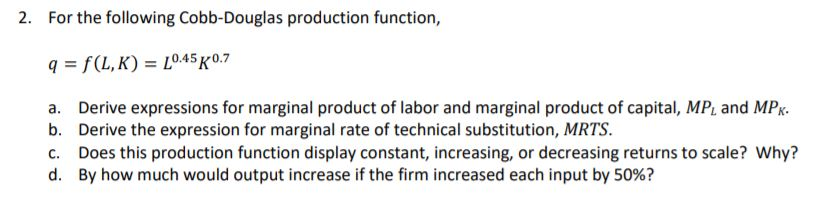2. For the following Cobb-Douglas production function, q = f(L,K) = _0.45 0.7 a. Derive expressions for marginal product of labor and marginal product of capital, MP, and MPK. b. Derive the expression for marginal rate of technical substitution, MRTS. C. Does this production function display constant, increasing, or decreasing returns to scale? Why? d. By how much would output increase if the firm increased each input by 50%?

• ### SHOW ALL WORK!!! 2. For the following Cobb-Douglas production function, q=f(L,K) = _0.45 0.7 a. Derive expressions for m...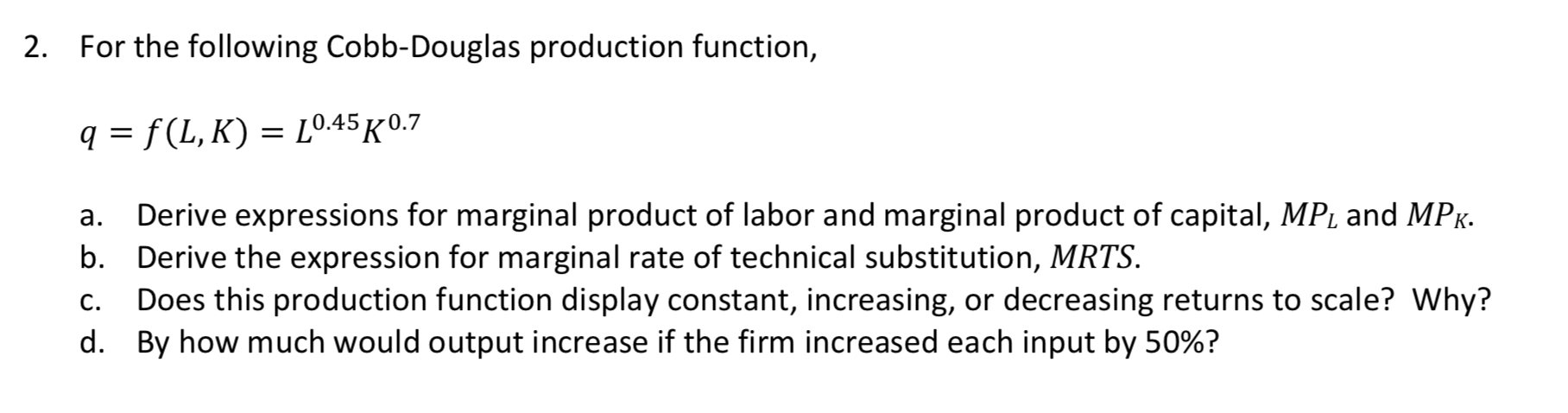SHOW ALL WORK!!! 2. For the following Cobb-Douglas production function, q=f(L,K) = _0.45 0.7 a. Derive expressions for marginal product of labor and marginal product of capital, MP, and MPK. b. Derive the expression for marginal rate of technical substitution, MRTS. C. Does this production function display constant, increasing, or decreasing returns to scale? Why? d. By how much would output increase if the firm increased each input by 50%?

• ### Question 4 a) The firm ACME has the production function f ( K , L)=K 2...

Question 4 a) The firm ACME has the production function f ( K , L)=K 2 3 L 2 3 . Calculate an expression for the marginal product of labour, L , and establish if it is increasing, constant or decreasing. Verify if ACME’s production technology exhibits diminishing, constant or increasing returns to scale. (6p) b) Set up ACME’s long run profit maximization problem and derive the factor demands for optimal choice of y. Question 5 (Credit question) Try to...

Free Homework App

Scan Your Homework
to Get Instant Free Answers
Need Online Homework Help?

Get Answers For Free
Most questions answered within 3 hours.